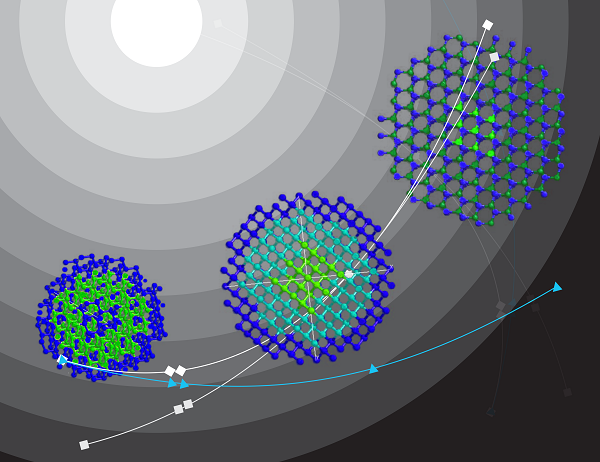﻿

Basic Structure of Hindi Poetry
Part 2a - Software "Geet Gatiroop" to help in Maatraa Counting

Vinod Tewary and Vani Murarka
(Editors, Kaavyaalaya.org)

Preface
Part 2 of the article series on the Basic Structure of Hindi poetry dealt with an introduction of Maatraa (मात्रा) in metered (छन्दोबद्ध) poems. Subsequent to the publication of that article, a software is now available, which can assist you in maatraa counting. This addendum to Part 2, illustrates the same examples of Part 2, this time using the software. This hopefully will enable a further understanding of those examples as well as serve as an introduction for how to the software can help you in applying the principles laid down in Part 2.

The whole article is in 4 parts as listed below:

Part 1: Structural units of a poem.
Part 2: Maatraa (मात्रा) or Meter of a Poem for Chhanddobaddh (छन्दोबद्ध) Poetry - i.e. poems that follow a clear rhyme and rhythm. This is perhaps the most important ingredients of the craft of poetry.
Part 2a: (This part) Software “Geet Gatiroop” (गीत-गतिरूप) to help in Maatraa Counting
Part 3: Rhythm in Mukt-Kavita (free verse) based on Maatraa and other structures.
Part 3a: Geet Gatiroop software for Mukt-Kavita
Part 4: Correspondence with Urdu poetry

1. Introduction
The software Geet-Gatiroop ( गीत-गतिरूप ) provides a visual output of the maatraa arrangement of a given set of lines of poetry, in the same way as the examples in Part 2 were illustrated with diagrams. Thus, for example, the lines

उमा कहउं मैं अनुभव अपना
सत हरि भजन जगत सब सपना

 उमा(1+2) कहउं(1+1+1) मैं(2) अनुभव(1+1+1+1) अपना(1+1+2) = total 16 सत(1+1) हरि(1+1) भजन(1+1+1) जगत(1+1+1) सब(1+1) सपना(1+1+2) = total 16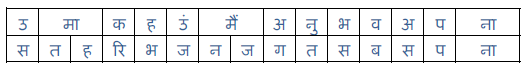Example from Part 2

is displayed by the software as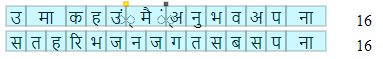This software encodes the principles laid out in Part 2 that are largely applicable to any Hindi text – i.e. the fact that laghu swar (short vowels) occupy 1 maatraa, deergh swar (long vowels) occupy 2 maatraas, and the generally applicable rules of when when half-letters consume a maatraa and when it does not. However, the software cannot take care of exception cases, and other finer adjustments that may be required for the specific text in question. That is something that only you can do. Thus, it is still important to equip oneself with the knowledge in the other parts of this article series, and use the software only as an assistant, a helper.

We shall now see how the same examples taken in Part 2, are handled by the software and what is required of you as a user.

2. Examples of Part 2, Re-seen via Geet Gatiroop

It does not need to be downloaded and installed. It is to be used at this address in the browser itself after you signup.

This is the software screen –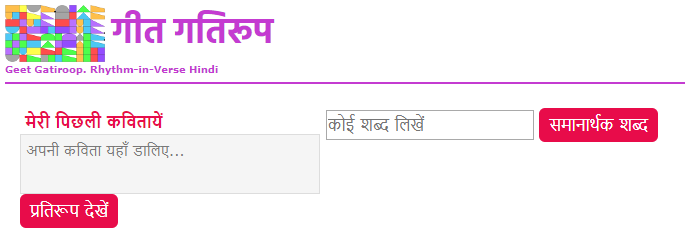To obtain the outputs as above, copy-paste (or type) your Hindi text in the box on the left and press the “प्रतिरूप देखें” button (or “Visualize” button for the English interface).

2 and 2 alignment
It is important to note that 1+2 cannot match with 2+1. However 2 can match with 1+1 so 1+2 or 2+1 can both match 1+1+1. For example " राह " (2+1) will not match with " रहा " (1+2) but both will match with " सड़क ".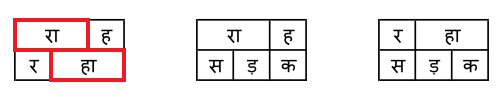Output from Geet-Gatiroop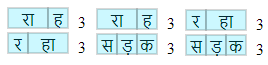The software has the facility to hide the text, which makes the maatraa alignment even more clear.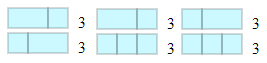Chaupaayee by Tulsidas उमा कहउं मैं अनुभव अपना

 उमा(1+2) कहउं(1+1+1) मैं(2) अनुभव(1+1+1+1) अपना(1+1+2) = total 16 सत(1+1) हरि(1+1) भजन(1+1+1) जगत(1+1+1) सब(1+1) सपना(1+1+2) = total 16First output from Geet-Gatiroop: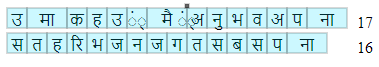The software has assigned one maatraa to the nasal sound (ं) at the end of कहउं. To correct that, click in that relevant box, so that it may contract to consume no maatraa. It is now shown as a small yellow-orange square.

Final output from Geet-Gatiroop:Short pronunciation of Deergh Swar (long vowel): Dohaa from Raamcharitmaanas

Dohaas are 24 maatraa. Consider an example of a doha in Raamcharitmaanas:
 बोले(2+2) बिहंसि(1+1+1) महेश(1+2+1) तब,(1+1) ज्ञानी(2+2) मूढ़(2+1) न(1) कोय(2+1) = 24 जो(2) जस(1+1) जब(1+1) हरिहर(1+1+1+1) चहंहि,(1+1+1) सो(2) तस(1+1) तेहि(1+1) क्षण(1+1) होय(2+1) = 24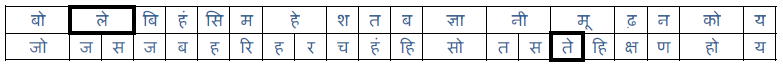Note that the maatraa of ले in ‘बोले’ in the first line is 2 whereas the maatraa of ते in तेहि is 1. This is apparent from their pronunciation. The letter ‘le’ in ‘bole’ is pronounced elongated whereas ‘te’ in ‘tehi’ is pronounced in short.

First output from software: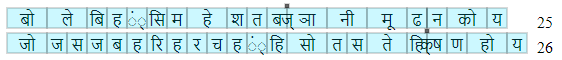Nasal sound in बिहंसि and चहंहि needs to be adjusted, “ते” in तेहि क्षण needs to be adjusted by clicking on the appropriate boxes. ते now is one maatraa wide, and yellow in color indicating that it was adjusted by the user. The nasal sounds are small yellow squares.

Final output from software: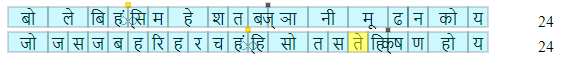Now let us take one more example, in which the rule of maatraa alignment is compromised, rather manipulated, by adding ‘lahraa’ or by elongating a letter or by giving a pause:

 कामिहि(2+1+1) नारि(2+1) पियारि(1+2+1) जिमि,(1+1) लोभिहि(2+1+1) प्रिय(1+1) जिमि(1+1) दाम(2+1) = 24 तिमि(1+1) रघुनाथ(1+1+2+1) निरंतर –अ -(1+1+1+1+1+1) प्रिय(1+1) लागहु(2+1+1) मोहि(1+1) राम(2+1) = 24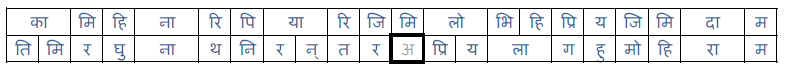The extra letter अ at the end of nirantar is a kind of lahra that elongates the last letter ‘र’. You can also pronounce it as ‘निरन्तरः’ or ‘निरन्तरा’ to get the same effect.

First output from the software: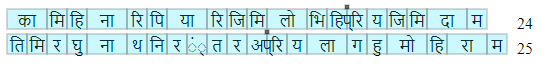Final output, after adjusting nasal sound of निरंतर –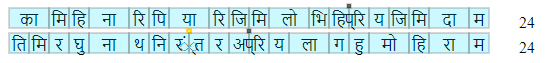You might wonder: Why does the software assign a maatraa to the nasal sound (ं), that you the user must remove always? The thing is that the (ं) can also indicate a half-letter that does indeed consume one maatraa, such as in the word जंगल which indeed is a 4 maatraa word. The (ं) in this case indicates the half letter ङ which consumes 1 maatraa.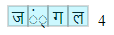Sthaayee from Jhansi Kee Rani

 बुंदेले(1+1+2+2) हरबोलों(1+1+2+2) के(2) मुँह(1+1) हमने(1+1+2) सुनी(1+2) कहानी(1+2+2) थी,(2) = 30 खूब(2+1) लड़ी(1+2) मर्दानी(1+1+2+2) वह(1+1) तो(2) झाँसी(2+2) वाली(2+2) रानी(2+2) थी(2) = 30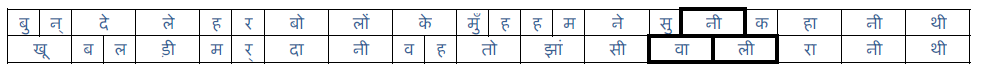Output from Geet-Gatiroop: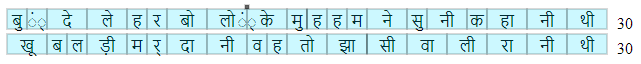Antara from Jhansi Kee Rani

 जाओ(2+2) रानी(2+2) याद(2+1) रखेंगे(1+2+2) हम(1+1) कृतज्ञ(1+1+1+1) भारतवासी,(2+1+1+2+2) = 30 यह(1+1) तेरा(2+2) बलिदान(1+1+2+1) जगावेगा(1+2+2+2) स्वतंत्रता(1+1+1+1+2) अविनाशी,(1+1+12+2) = 30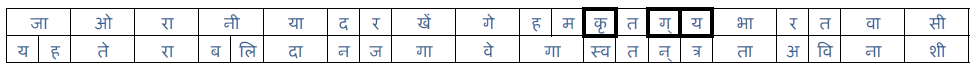First output: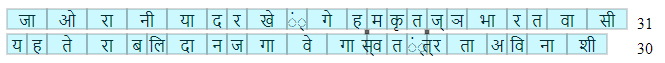Final output after adjusting the nasal sound in रखेंगे: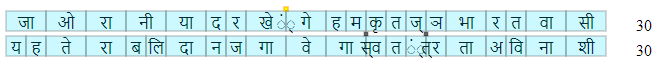Urdu ghazal from Shakeel

 बेज़ुबानी(2+1+2+2) जुबाँ(1+2) न(1) हो(2) जाये(2+2) = 17 राज़े-उलफ़त(2+1+1+1+1+1) अयाँ(1+2) न(1) हो(2) जाये(2+2) = 17

This is matlaa. Notice both the lines rhyme as 'jaaye'. The number of maatraas in each line is 17. The maatraa structure is same- if there is 2 in the top line, the bottom line at that place is either 2 or 1+1. Notice that ए in बेज़ुबानी is counted as 2, whereas in राज़े it is counted as 1 because it has a long pronunciation in bezubaanee and a short pronounciation in raaze. In general in composite possessive nouns in urdu, ए has a short pronunciation and has 1 maatraa (For example - गमे-दिल). Here is another couplet in the same ghazal.

 ज़िक्र(1+1+1) उनका(1+1+2) जुबान(1+2+1) पर(1+1) आया(2+2) = 17 यह(1+1) कहीं(1+2) दास्ताँ(2+1+2) न(1) हो(2) जाये(2+2) = 17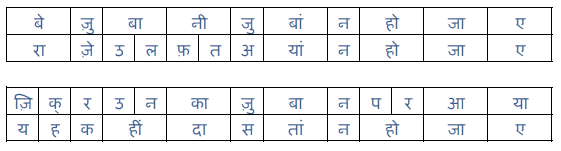First output -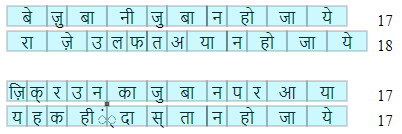Output by adjusting जे in राजे -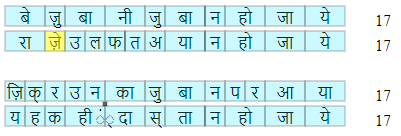Three videos are available, which explain the principles of Part 2 in a more visual manner with the use of the Geet-Gatiroop software. We recommend that you see the videos. They will enable you to further understand the concepts and know how the software can be used.

Next, Part 3: Rhythm in Mukt-Kavita (free verse) based on Maatraa and other structures.

10th August 2013
Modified on 19 December 2019. Images were updated to reflect the latest output from Geet Gatiroop.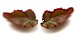इस महीने :
'पावस गीत'
प्रभात कुमार त्यागी

पुल बारिश का!
बिना ओढ़नी हवा घूमती
सबने देखा
पुल बारिश का!

मेघों से धरती तक
धानखेती सीढ़ियाँ,
मिट्टी में उग रहीं
नई हरी पीढ़ियाँ,
उड़ती हुई नदी पर
बनती मिटती नौका,
पुल बारिश का!
..

पूरी प्रस्तुति यहाँ पढें और सुनें...

डूब कर देखो ये है गंगा गणित विज्ञान की।
ये परम आनंद है वाणी स्वयं भगवान की।

~ विनोद तिवारी